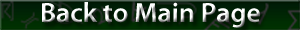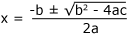Basic Mathematical Laws and Formulas

#### Basic Mathematical Laws and Formulas

Name Description Notes
Commutative law a + b = b + a
a × b = b × a
Quadratic formulaSolution for the general quadratic equation: ax2 + bx + c = 0